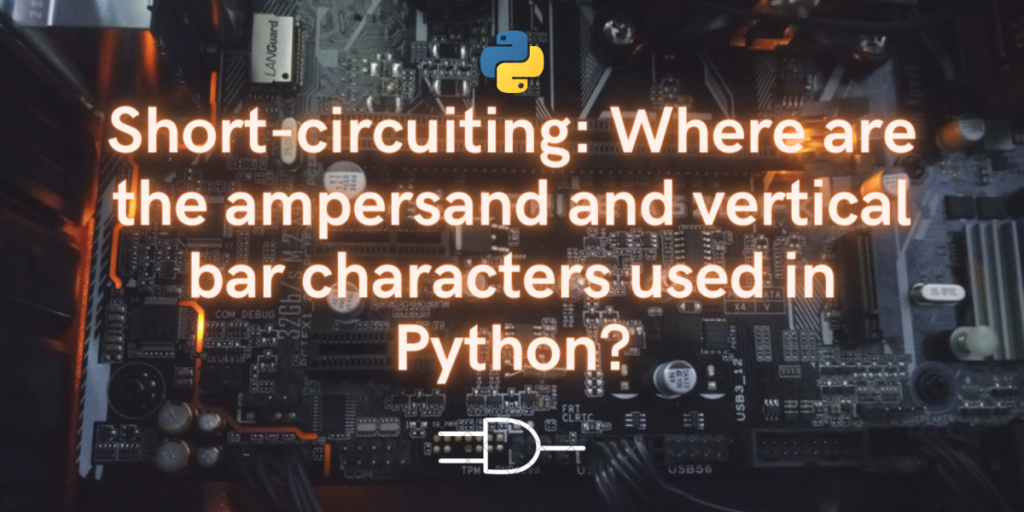# What is Short Circuiting in Python: Ampersand (&) & Vertical Bar (|)In this tutorial, we will go through what short-circuiting is in Python and how can we use it in our code. We’ll also find out how the ampersand symbol and the vertical bar character can be used in Python for performing selected operations.

Short circuiting in programming refers to the compiler or interpreter skipping over some expressions in a condition or a logical statement because the requirement has already been met. In other words, if the value of a variable or an expression has been pre-determined before checking particular conditions, it is called short circuit.

Short circuiting is carried out using the logical statements, namely, “AND”, “OR” and “NOT”. All of these logical operators, when used inside expressions return boolean values, “TRUE” or “FALSE”.

In programming languages besides python, the AND, OR and NOT operators are represented by the “&&” symbol, “||”symbol and “!” symbols respectively. In python, logical operators are used as is – as per the name. However, the single ampersand “&” and the single vertical bar “|” is used to evaluate binary expressions. They can also be used for intersection and union in sets.

## Mastering Short Circuiting in Python

Using the three logical operators AND, OR and NOT, short circuiting is carried out in Python. These three expressions are used as logical operators which perform the same function as their corresponding logic gates.

We can demonstrate these logical gates, in the following way:

• OR gate: For example, if we write, A or B, B will be executed only if A turns out to be false. So if A is false, then B, else, if A is true, then B is not executed and the program then continues as is.
• AND gate: For example, if we write A and B, First the A statement will be executed and if and only if A is true. Else, if A is false, then B is not even evaluated and the code progresses as is.

In case of OR, only the first statement is evaluated in the beginning.

• If that is true, the second statement is skipped.
• If and only if the first statement is false, the second statement is evaluated.
• If any one of the statements is true, the end result is true.
• If and only if both statements are false, the ultimate boolean value returned is false.

In case of AND, the first statement is executed.

• If and only if the first statement or expression is true, the compiler moves over to the second statement and evaluates it.
• If the first statement is false, then the second statement is ignored by the compiler and the boolean value returned is false.
• If the first statement is true and the second one is false, then also the returned value is false.
• Both statements need to be true, for the returned value to be true.
```A = True
B = False

if (A and B):
#do something

if (A or B):
#do something
```

In comparison to other languages such as C,

```//c syntax
#include <stdio.h>
int main()
{
int x = 1, y = 0;

if (x || y) {   //or statement
printf("%d", x);
}
if (x &&y){   //and statement
printf("%d",y);
}

return 0;
}
```

Similar: Binary Numbers and Their Operations in Python – Complete Guide

## What Are Single Ampersand & Vertical Bars in Python

Single ampersands and vertical bars in python are called eager operators. They are used to check binary expressions. The boolean value “true” corresponds to 1 in binary and the value “false” corresponds to 0 in binary.

Let’s delve into an example showcasing the use of these bitwise operators in Python

```#using single vertical bar
bin(1 | 0)
#using single ampersand
bin(0 & 1)
```

In the first statement, we take one true and the second false boolean value and use the singular or operator. Whereas, in the second line, we are using the binary ampersand operator.

The output gives us true in the first case as explained above and in the second one it’s false.

```0b1 #true
0b0 #false
```

We can also use this as intersection and union operators on python sets. When the binary OR operator is used, it gives us the union of both sets. And when we use the binary ampersand, it gives us the intersection of two sets.

```#intersection and union of sets
s1= set("xyzw")
s2= set("spyz")
s2 & s1
s2 | s1
```

The output will be:

```{'y', 'z'} #intersection of s1 and s2
{'p', 's', 'w', 'x', 'y', 'z'} #union of s1 and s2
```